# Short Answers Type Questions: Statistics - Mathematics (Maths) Class 9

Question 1. Find the mean of: 10, 90, 20, 80, 30 and 70.
Solution:
Number of observations = 6 Sum of the observations = 10 + 90 + 20 + 80 + 30 + 70 = 300
∴ Mean = (300/6)= 50
Thus, the required mean = 50

Question 2. Find the range and prepare a frequency table for the following observations:
4, 1, 1, 2, 3, 5, 2, 3, 3, 1, 2, 2, 4, 2, 5, 4, 1, 1, 3, 2
Solution:
Arranging the observations in an ascending order:
1, 1, 1, 1, 1, 2, 2, 2, 2, 2, 2, 3, 3, 3, 3, 4, 4, 4, 5, 5
Lowest observation = 1
Highest observation = 5

∴ Range = 5 – 1 = 4
Thus, the required range = 4.
Frequency table:

 Observation Tally marks Frequency 1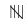5 2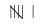6 3 IIII 4 4 III 3 5 II 2

Question 3. Make a cumulative frequency table for the following:

 Class interval Tally marks Frequency 100-110 IIII 4 110-1206 120-130 II 2 130-140 III 3 140-1505 Total 20

Solution: Cumulative frequency table:

 Class interval Tally marks Frequency Cumulative frequency 100-110 IIII 4 4 110-1206 6 + 4 = 10 120-130 II 2 10 + 2 = 12 130-140 III 3 12 + 3 = 15 140-1505 15 + 5 = 20 Total 20

Question 4. Form a frequency table for the following:

 Marks obtained Number of students More than 50More than 40More than 30More than 20More than 10More than 0 02037444650

Solution:

 Marks obtained Frequency Cumulative frequency 0–1010–20  20–3030–40  40–50 50 – 46 = 4  46 – 44 = 2 44 – 37 = 7 37 – 20 = 17 20 – 0 = 20 46133050 Total 50

Question 5. Find the mean of the first six multiples of 6.
Solution:
Six multiples of 6 are: 6, 12, 18, 24, 30, 36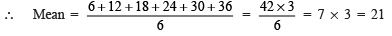Thus, the required mean = 21

Question 6. If the mean of 8, 5, 2, x, 6, 5 is 6, then find the value of x.
Solution:
We have the number of observations = 6

∴               Mean =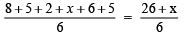Now,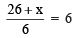[∵ Mean is 6]

⇒ 26 + x = 6 x 6 ⇒ x = 36 – 26 = 10

Question 7. If the mean of the following data is 15 then find the value of p.

 xi 5 10 15 20 25 fi 6 4 5 p 7

Solution: We can have the following table from the given data :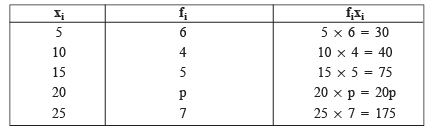∴ we have           ∑ (fi) = 6+4+5+p+7 = 22+p

∑ (xifi) = 30+40+75+20p+175 = 320+20p

∵ Mean,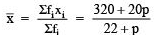But Mean = 15

∴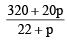⇒ 320 + 20p = 15(22 + p)
⇒ 320 + 20p = 330 + 15p
⇒ 20p – 15p = 330 – 320
⇒ 5p = 10
⇒   p= (10/5)= 2
Thus, the required value of p is 2

Question 8. If the mean of the following data is 18.75, find the value of p:

 xi 10 15 20 p 30 fi 5 10 7 8 2

Solution: From the given data we can prepare the following table:

 xi fi (fi * xi) 10 5 10 x 5 = 50 15 10 15 x 10 = 150 20 7 20 x 7 = 140 P S p x 8 = 8p 30 2 30 x 2 = 60 ∑(fi) = 32 ∑(xi * fi) = 400 + 8p

∵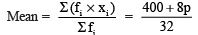But the mean is 18.75.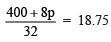⇒ 400 + 8p = 18.75 x 32
⇒ 400 + 8p = 600
⇒ 8p = 600 – 400 = 200
⇒   p= (200/8) = 25
Thus, the required value of p is 25.

Question 9. The following observations are arranged in ascending order:
26,  29,  42,  53,  x,  x + 2,  70,  75,  82,  93
If the median is 65, find the value of x.
Solution:
Number of observations (n) = 10 Here ‘n’ is even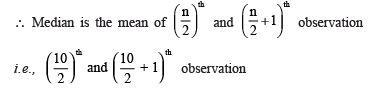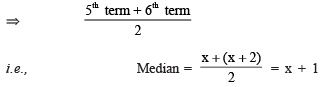∴ x + 1 = 65 or x = 65 – 1 = 64

Thus, the required value of x is 64.

Question 10. Construct a frequency table with equal class intervals from the following data on the monthly wages (in rupees) of 30 workers of a factory taking one of the class interval as 8440–8540 (8540 not included). 8740, 8780, 8760, 8740, 8450, 8200, 8440, 9080, 8880, 8840, 8340, 8140, 8660, 8960, 8400, 9100, 8460, 8880, 8540, 8140, 8760, 8300, 8350, 8660, 8950, 9120, 9100, 8320, 8150, 9080.
Solution:
Here, the lowest observation = 8140
The highest observation = 9120
∵ One of the classes is 8440–8540,
i.e. the class size = 100
∴ To cover the given data, we have the classes as: 8140–8240; 8240–8340; ….; 9040–9140.
Now, the required frequency table is:

 Wages (in f) Tally marks Frequency 8140-8240 IIII 4 8240-8340 II 2 8340-8440 III 3 8440-8540 III 3 8540-8640 I 1 8640-8740 II 2 8740-88405 8840-8940 III 3 8940-9040 III 3 9040-9140 IIII 4 Total 30

Question 11. The mean of 40 numbers was found to be 35. Later on, it was detected that a number 56 was misread as 16. Find the correct mean of the given numbers.
Solution:
Number of observations = 40
∵ Calculated mean = 35
∴ Calculated sum = 40 x 35 = 1400
Since the number 56 is misread as 16.
∴ Correct sum of the numbers = 1400 – [Wrong observation] + [Correct observation]
= 1400 –  + 
= (1400 – 16 + 56) = 1440
∴ The correct mean =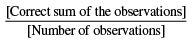= (14440/40)= 36
Thus, the correct mean = 36.

Question 12. The mean of 72 items was found to be 63. If two of the items were misread as 27 and 9 instead of 72 and 90 respectively. Find the correct mean.
Solution:
Number of items = 72
Calculated mean = 63
∴ Calculated sum = 72 x 63 = 4536
Since, the item 72 is misread as 27.
And the item 90 is misread as 9.
∴ Correct sum of 72 items = [Calculated sum] – [Sum of the wrong items] + [Sum of the correct items]
=  – [27 + 9] + [72 + 90] =  –  + 
= [4536 – 36 + 162] = 4662
∴ Correct mean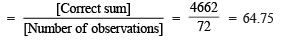Thus, the required correct mean is 64.75.

Question 13. A train travels between two stations x and y. While going from x to y, its average speed is 72 km per hour, and while coming back from y to x, its average speed is 63 km per hour. Find the average speed of the train during the whole journey.
Solution:
Let the distance between x and y is s km.
Since, average speed = Total Distance / Total Time

For the train going from x to y, Average speed = 72 km/hr
∴ Time taken = Distance / speed = s/72 hours

For the train coming back from y to x, Average speed = 63 km/hr
∴ Time taken = Distance / speed = s/63 hours

Now, the total time taken to cover 2s km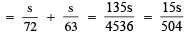∴ Average speed during the whole journey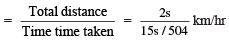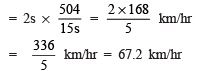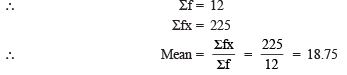Thus, the required average speed = 67.2 km/hr.

Question 14. Find the mode for the following data using the relation: mode = (3 median – 2 mean)

 Item (x) Frequency (f) 16 17 18 19 20 21 113412

 Item (x) Frequency (f) Cumulative frequency fx 161718192021 113412 12591012 161754762042 Total 225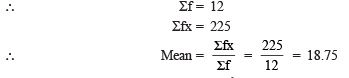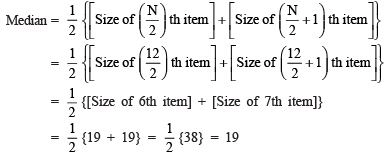Since, Mode = 3(Median) – 2(Mean)
∴ Mode of the above data = 3(19) – 2(18.75)
= 57 – 37.5 = 19.5

The document Short Answers Type Questions: Statistics | Mathematics (Maths) Class 9 is a part of the Class 9 Course Mathematics (Maths) Class 9.
All you need of Class 9 at this link: Class 9

 1. What is statistics?Ans. Statistics is a branch of mathematics that involves collecting, organizing, analyzing, interpreting, and presenting data. It helps in making informed decisions by providing valuable insights into various phenomena and patterns in the data.
 2. What are the two main types of statistics?Ans. The two main types of statistics are descriptive statistics and inferential statistics. Descriptive statistics involves summarizing and describing the main features of a dataset, such as measures of central tendency (mean, median, mode) and measures of dispersion (range, variance, standard deviation). Inferential statistics, on the other hand, involves making inferences or predictions about a population based on a sample.
 3. What is the importance of statistics in everyday life?Ans. Statistics plays a crucial role in everyday life. It helps in understanding and interpreting data, making informed decisions, and solving real-life problems. For example, statistics is used in determining the average rainfall in a region, predicting stock market trends, analyzing survey data, and studying the effectiveness of medical treatments.
 4. What is the difference between qualitative and quantitative data?Ans. Qualitative data refers to non-numerical information that describes qualities or characteristics. It is typically obtained through observations, interviews, or open-ended survey questions. On the other hand, quantitative data refers to numerical information that can be measured or counted. It is obtained through methods such as surveys, experiments, or measurements. The main difference between the two is that qualitative data is subjective and categorical, while quantitative data is objective and numerical.
 5. How is statistics used in research?Ans. Statistics is extensively used in research to analyze and interpret data. It helps researchers make sense of the data they collect and draw meaningful conclusions. Statistics is used in designing research studies, selecting appropriate sample sizes, analyzing data using various statistical tests, and drawing conclusions based on the results. It allows researchers to determine the significance of their findings and make generalizations about the population they are studying.

## Mathematics (Maths) Class 9

62 videos|426 docs|102 tests

## Mathematics (Maths) Class 9

62 videos|426 docs|102 tests
Signup to see your scores go up within 7 days! Learn & Practice with 1000+ FREE Notes, Videos & Tests.
10M+ students study on EduRev
Track your progress, build streaks, highlight & save important lessons and more!(Scan QR code)
Related Searches

,

,

,

,

,

,

,

,

,

,

,

,

,

,

,

,

,

,

,

,

,

;